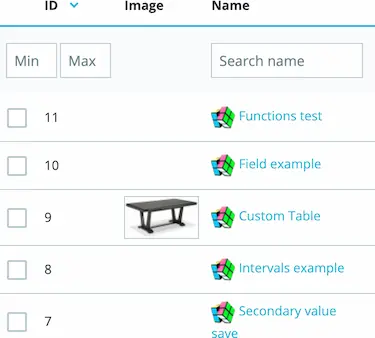# # PHP Calculation

If your calculation is very complex or requires custom logic, then you can use a PHP file to do it. PHP can help you in these situations for example:

• Your calculation is too complex to be written in a formula
• You want to access a PrestaShop variable that is not available in the formula
• You want to fetch data from a remote service

Here are the available methods to use a PHP file:

## # Accessing fields from PHP

In any PHP file that you create inside the folder `/dynamicproduct`, the fields will be available with their current values. For example, if you have fields `width`, `length` and `height`, you can access them using the variables `\$width`, `\$length` and `\$height` respectively.
In the same way, you can change a field value by directly assigning a new value to the corresponding variable, for example:

``````\$width = 10;
\$length = 15;
\$text = 'Hello';
``````

Additionally, the module provides a variable named `\$changed` which indicates the name of the field that was changed by the customer.
This can be useful if you want to only update the field that wasn't changed when two fields depend on each other.

## # Accessing PrestaShop functions

In the calculation file, you can access any PrestaShop function as well, here are some examples:

``````// fetch a file from a remote service
\$content = Tools::file_get_contents('http://google.com');

// get the group of the current customer
\$id_group = Context::getContext()->customer->id_default_group;

// get the category id of the current product
\$id_category = (new Product(\$id_product))->id_category_default;
``````

## # Other available variables

In addition to the fields, the module provides other variables in the PHP context:

``````// The ID of the current product
/** @var int \$id_product */

// The selected combination ID
/** @var int \$id_attribute */

// The current selected quantity
/** @var int \$quantity */

// The product base price
/** @var float \$product_price */

// The product base weight
/** @var float \$product_weight */

// The name of the field that the customer changed to trigger the calculation
/** @var string \$changed */
``````

TIP

You can copy the above code to help you with autocompletion

## # Assigning a value to a field (Field Allocation)

You can assign a value to field dynamically using PHP.
First, create a php file in the folder `/dynamicproduct/allocations`

• Name the file `products.php` if you want to target all products
• Name the file `productX.php` if you want to target only the product with ID=X

You can access fields by name and you can also assign a value to fields by name, here are some examples:

• Assign a value to an area field (this can be done using Field formulas but it's here only as an example)
``````// Assuming you have fields named area, width and length
\$area = \$width * \$length;
``````
• Assign a PrestaShop variable or state to a field
``````// Assuming you have a field named logged_in
\$logged_in = (int)Context::getContext()->customer->isLogged();
// This will return 1 or 0
// It can be used to show/hide fields using the conditions feature for example
``````

TIP

Don't forget to create a dynamic variable field before assigning its value

Once you assign a value to a field, you can use it in the price formula and all other formulas and functions such as the intervals, the conditions and the grids etc...

## # Price calculation using PHP

To calculate the price, place a PHP file in the folder `/dynamicproduct/calculator`

• Name the file `products.php` if you want to target all products

• Name the file `productX.php` if you want to target only the product with ID=X
For example:
`product1.php` will target product `#1`
`product42.php` will target product `#42`
The product ID is displayed in the product list in the backofficeIn the price calculation, you need to assign a `\$result` variable at the end of the calculation.

TIP

Initially, the `\$result` variable will contain the price formula result

``````\$result = \$width * \$length / 10000 * 50;
``````

Examples:

• Apply a discount when width is bigger than 100
``````if (\$width > 100) {
// We don't assign a new value in this case, we simply reduce the price formula result
\$result = \$result * 0.8;
}
``````
• Get a unit price from a remote service
``````\$unit_price = (float)Tools::file_get_contents('https://remoteservice.com/pricing.php');
\$result = \$length * \$unit_price;
``````

## # Weight calculation using PHP

The weight calculation is done exactly in the same way as the price calculation, except for two differences.

• The variable that has to be assigned is called `\$weight`
• The file names are `weights.php` and `weightX.php` to target a product with ID=X

Here's an example

``````// Calculate the weight based on the volume
\$weight = \$width * \$length * \$depth * 5;
``````

TIP

Initially, the `\$weight` variable will contain the weight formula result

## # Quantity calculation using PHP

The quantity calculation is also very similar to the price calculation, but here's the two differences:

• The variable that you need to assign is called `\$qty`
• The file names are `quantity.php` and `quantityX.php` to target a product with ID=X
Here are some examples:
``````// Retract from the stock by meter squared instead of units
\$qty = \$width * \$length;
``````

TIP

Initially, the `\$qty` variable will contain the quantity formula result

## # Declaring JavaScript variables from PHP

The module allows you to declare JavaScript variables that you can use in your custom scripts.
First, create a php file in the folder `/dynamicproduct/declarations`

• Name the file `products.php` if you want to target all products
• Name the file `productX.php` if you want to target only the product with ID=X

The variables that you need to assign is called `\$declarations`, it's a PHP array.

Here's a code example

``````\$declarations = array(
'id_category' => (new Product(\$id_product))->id_category_default,
'id_group' => Context::getContext()->customer->id_default_group,
);
``````

These variables can be then accessed from JavaScript like this

``````window.id_category
window.id_group
``````
Last Updated: 4/21/2021, 12:37:35 PM